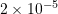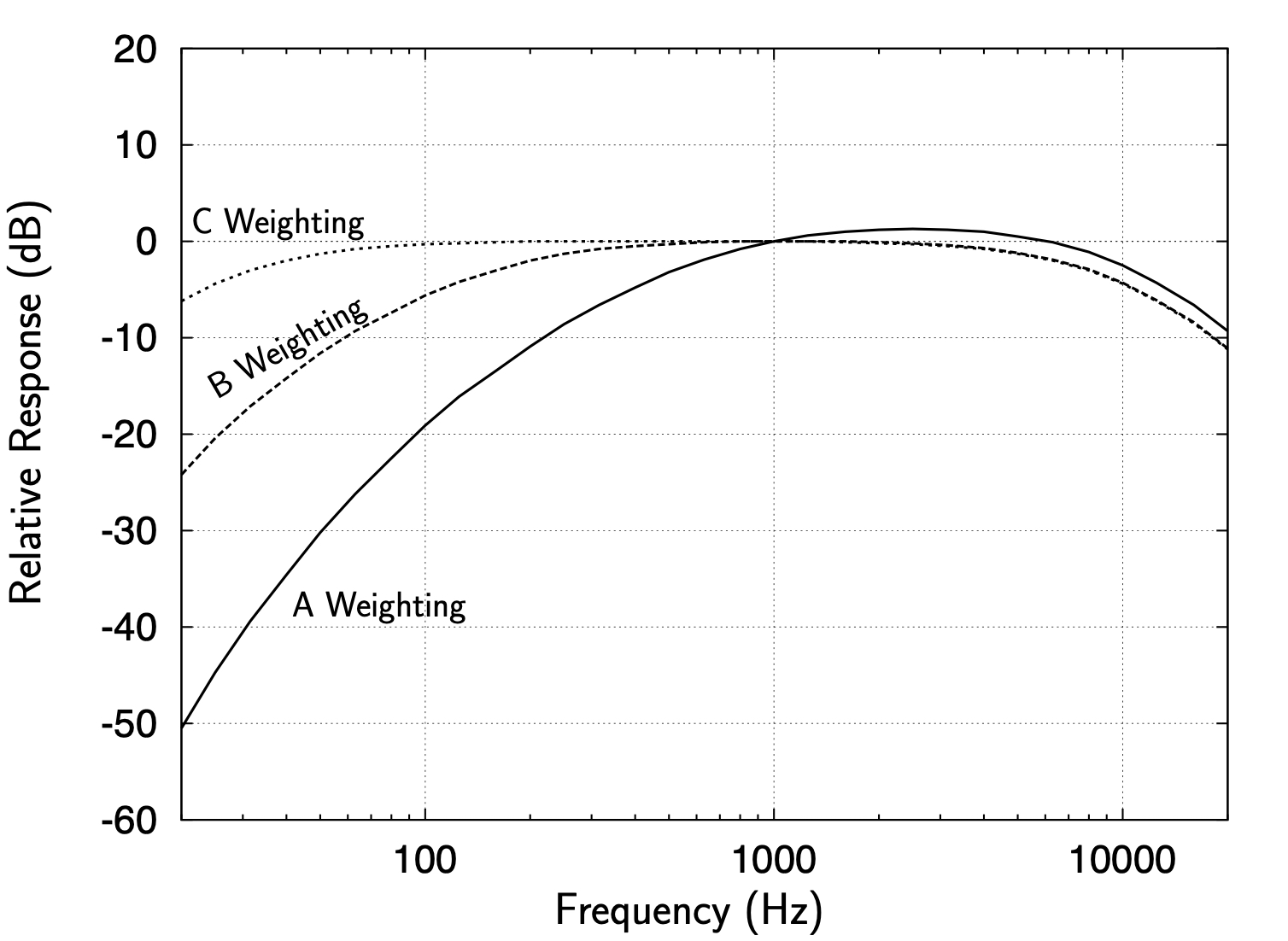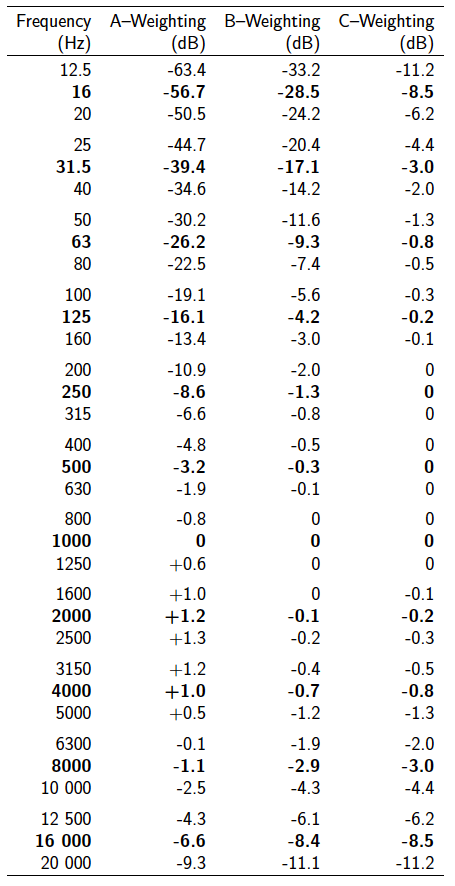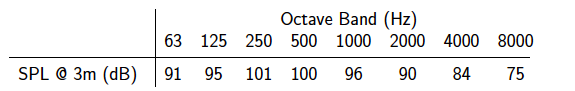### Sound and Acoustics: Psychoacoustics and Weighting Networks

Often in acoustics the true (RMS) sound pressure levels that are measured must be modified or weighted, typically depending on their frequency. This is done as a result of regulatory requirements. The reason for this is that most acoustic requirements are based on human hearing and we do not respond the same to sounds at different frequencies. For example, the threshold of hearing (Pa) discussed previously was for sounds in the 1000-2000 HZ range. This is the most sensitive region of human hearing. (Most of the frequency content in human speech is in the 1000–4000 HZ range.) At lower frequencies, the hearing threshold is higher. In fact, human hearing sensitivity falls off very rapidly below 1000 HZ and above approximately 10 000 HZ. The study of how we respond to sound is known as psychoacoustics.

As a result of this frequency dependence of the human hearing response, weighting scales are used to alter the spectral characteristics of noise. This is done typically to better represent how well a given noise can be tolerated. Frequencies to which we are more sensitive get a higher weighting while those to which we are less sensitive get a lower weighting. In practice, a weighting scale is simply a table of corrections which is applied to sound pressure levels as a function of frequency.

There have been many weighting scales developed and used. Some important ones are discussed below.

•  A–Weighting Scale.
The most common weighting scale in acoustics is the A–weighting scale. This is the one which most closely approximates the frequency response of the human ear to sound. The rates of hearing loss tend to follow the A scale as well. One can tolerate higher levels of low frequency noise for a longer period without impairment. For these reasons the A–weighting scale is by far the most predominant in noise measurement and control. Most electronic sound measurement equipment are able to determine A–weighted sound pressure levels directly.
• C–Weighting Scale.
The C–weighting scale is relatively flat in the frequency range most often of interest and as such is useful in providing a baseline reference for comparison.
• Linear Weighting.
Note that a sound pressure level measurement with no weighting adjustments in known as a linear weighting.Figure 12.11: Sound pressure level corrections for several weighting scales

Figure 12.11 illustrates the corrections used for several weighting scales. Corrections used for the standard octave scales are shown in Table 12.7Table 12.7: Weighting Scale Corrections for Octave and One-Third Octave Bands

#### Example

The octave band sound pressure levels (unweighted) for a centrifugal fan measured at 3 m are shown below.

1.Determine the overall noise level (SPL) at 3 m (unweighted).
2. Determine the overall noise levels using the
a) A-Weighting scale
b) C-Weighting scale